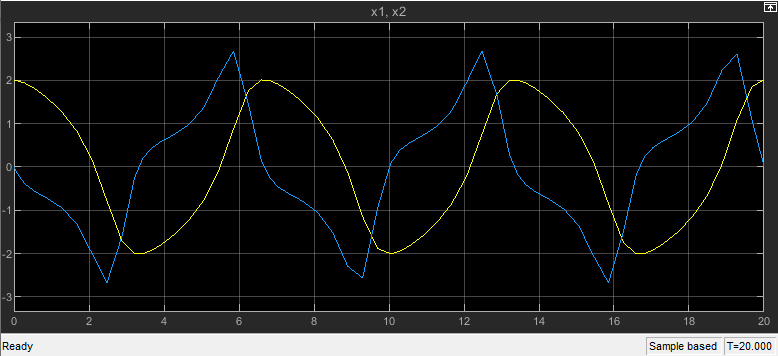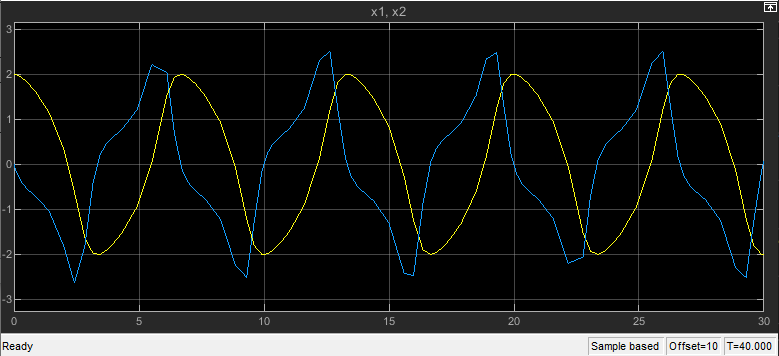# Stop time

Simulation stop time

Model Configuration Pane: Solver

## Description

Specify the stop time for the simulation or generated code in seconds, as a double-precision value.

## Settings

scalar

Default: `10`

• The stop time must be greater than or equal to the start time.

• Specify `inf` to run a simulation or generated program until you explicitly pause or stop it.

• If the stop time is the same as the start time, the simulation or generated program runs for one step.

• Simulation time is not the same as clock time. For example, running a simulation for 10 seconds usually does not take 10 seconds. Total simulation time depends on many factors, such as model complexity, solver step size, and system speed.

• If your model includes blocks that depend on absolute time and your design runs indefinitely, see Blocks That Depend on Absolute Time.

## Examples

expand all

Open the model `vdp`.

```mdl = "vdp"; open_system(mdl)```

The model is saved with a start time of `0` seconds and a stop time of `20` seconds.

`get_param(mdl,"StartTime")`
```ans = '0.0' ```
`get_param(mdl,"StopTime")`
```ans = '20' ```

Simulate the model. To view the simulation results, double-click the Scope block. The Scope window displays the results from the start time to the stop time.

`out1 = sim(mdl);`Change the start time to `10` seconds and the stop time to `40` seconds.

1. On the Modeling tab, under Setup, click Model Settings.

2. Select the Solver pane.

3. In the Start time box, enter `10`.

4. In the Stop time box, enter `40`.

5. Click OK.

Alternatively, use the `set_param` function to configure the start and stop time programmatically.

`set_param(mdl,"StartTime","10","StopTime","40")`

Simulate the model again. The Scope window updates to reflect the longer simulation time. The time axis ranges from 0 to 30, with a 10-second offset indicated in the lower-right of the Scope window.

`out2 = sim(mdl);`To change the start and stop time for a simulation without modifying configuration parameter values saved in a model, use a `Simulink.SimulationInput` object.

Open the model `vdp`.

```mdl = "vdp"; open_system(mdl)```

As saved, the model has a start time of `0` seconds and a start time of `20` seconds.

`get_param(mdl,"StartTime")`
```ans = '0.0' ```
`get_param(mdl,"StopTime")`
```ans = '20' ```

Create a `Simulink.SimulationInput` object to configure a simulation of the model.

`simIn = Simulink.SimulationInput(mdl);`

Use the `setModelParameter` function to specify a start time of `10` seconds and a stop time of `40` seconds for the simulation.

```simIn = setModelParameter(simIn,"StartTime","10",... "StopTime","40");```

Simulate the model using the `SimulationInput` object.

`out = sim(simIn);`

The simulation uses the start time and stop time values defined on the `SimulationInput` object.

`tFirst = out.yout{1}.Values.Time(1)`
```tFirst = 10 ```
`tLast = out.yout{1}.Values.Time(end)`
```tLast = 40 ```

The configuration parameter values in the model remain unchanged.

`get_param(mdl,"StartTime")`
```ans = '0.0' ```
`get_param(mdl,"StopTime")`
```ans = '20' ```

## Recommended Settings

The table summarizes recommended values for this parameter based on considerations related to code generation.

ApplicationSetting
DebuggingNo impact
TraceabilityNo impact
EfficiencyNo impact
Safety precautionA positive value

## Programmatic Use

 Parameter: `StopTime` Type: string | character vector Values: double Default: `'10.0'`

## Version History

Introduced before R2006a